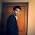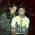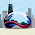# GBM (Boosted Models) Tuning Parameters

In Stochastic Gradient Boosting Tree models, we need to fine tune several parameters such as n.trees, interaction.depth, shrinkage and n.minobsinnode (R gbm package terms).

The detailed explanation is as follows -

1. n.trees – Number of trees (the number of gradient boosting iteration) i.e. N. Increasing N reduces the error on training set, but setting it too high may lead to over-fitting.

2. interaction.depth (Maximum nodes per tree) - number of splits it has to perform on a tree (starting from a single node).
More than two nodes are required to detect interactions and the default six - node tree appears to do an excellent job
interaction.depth = 1 : additive model, interaction.depth = 2 : two-way interactions, etc.

As each split increases the total number of nodes by 3 and number of terminal nodes by 2, the total number of nodes in the tree will be 3∗N+1 and the number of terminal nodes 2∗N+1
Salford Default Setting : 6 - node tree appears to do an excellent job
3. Shrinkage (Learning Rate) – It is considered as a learning rate.

Shrinkage is commonly used in ridge regression where it reduces regression coefficients to zero and, thus, reduces the impact of potentially unstable regression coefficients.

In the context of GBMs, shrinkage is used for reducing, or shrinking, the impact of each additional fitted base-learner (tree). It reduces the size of incremental steps and thus penalizes the importance of each consecutive iteration. The intuition behind this technique is that it is better to improve a model by taking many small steps than by taking fewer large steps. If one of the boosting iterations turns out to be erroneous, its negative impact can be easily corrected in subsequent steps.
Salford Default value = max(0.01, 0.1*min(1, nl/10000))
where nl = number of LEARN records.
This default uses very slow learn rates for small data sets and uses 0.1 for all data sets with more than 10,000 records.
High learn rates and especially values close to 1.0 typically result in overfit models with poor performance.  Values much smaller than .01 significantly slow down the learning process and might be reserved for overnight runs.
Use a small shrinkage (slow learn rate) when growing many trees.
One typically chooses the shrinkage parameter beforehand and varies the number of iterations (trees) N with respect to the chosen shrinkage.

4. n.minobsinnode - the minimum number of observations in trees' terminal nodes. Set n.minobsinnode = 10. When working with small training samples it may be vital to lower this setting to five or even three.

5. bag.fraction (Subsampling fraction) - the fraction of the training set observations randomly selected to propose the next tree in the expansion. In this case, it adopts stochastic gradient boosting strategy. By default, it is 0.5. That is half of the training sample at each iteration. You can use fraction greater than 0.5 if training sample is small.
Friedman showed that a subsampling trick can greatly improve predictive performance while simultaneously reduce computation time.
6. train.fraction - The first train.fraction * nrows(data) observations are used to fit the gbm and the remainder are used for computing out-of-sample estimates of the loss function (like out of bag error in random forest). By default, it is 1.

Important Note I : You can ignore step 5 and 6 to fine tune the GBM model.

Important Note II : Small shrinkage generally gives a better result, but at the expense of more iterations (number of trees) required.

Examples -
distribution = "bernoulli", n.trees = 1000, interaction.depth =6, shrinkage = 0.1 and n.minobsinnode = 10
distribution = "bernoulli", n.trees = 3000, interaction.depth =6, shrinkage = 0.01 and n.minobsinnode = 10

R Code : TreeNet (Gradient Boosting Tree)

1. Model Build
gbm1 = gbm(gb ~ ., data = german_data, distribution = "bernoulli", bag.fraction = 0.5, n.trees = 1000, interaction.depth =6, shrinkage = 0.1, n.minobsinnode = 10)
Important Point : Make sure the dependent variable is not defined as a factor if the dependent variable is binary. If it is a factor, multinomial is assumed. If the response has only 2 unique values (0/1), bernoulli is assumed; otherwise, if the response has class "Surv", coxph is assumed; otherwise, gaussian is assumed.

2. Variable Importance
importance = summary.gbm(gbm1, plotit=TRUE)Related PostsDeepanshu founded ListenData with a simple objective - Make analytics easy to understand and follow. He has over 8 years of experience in data science. During his tenure, he has worked with global clients in various domains like Banking, Insurance, Telecom and Human Resource.

13 Responses to "GBM (Boosted Models) Tuning Parameters"
1.Thank you. This was very useful

1.Glad you found it useful. Cheers!

2.This was very helpful and detailed! Thanks!

1.Thank you for your lovely words.

3.I tried running the GBM using the above code like below
gbm1 = gbm(Status ~ .-Year-unique_eid, data = data_train, distribution = "bernoulli", bag.fraction = 0.5, n.trees = 1000, interaction.depth =6, shrinkage = 0.1,
n.minobsinnode = 10,verbose = TRUE)

but i am getting nan as output ,could you suggest where i am going wrong

Thanks,
Sandeep

1.Make sure the dependent variable is not defined as a factor if the dependent variable is binary. Convert it to character or numeric type.

4.Awesome, thankyou so very much for the clear explanation!

1.5.Thank you for doing this! I do have a few questions, though a little further down the rabbit hole than this post planned on addressing.

I'm using gbm.step in R and am unsure about some details of methodology. Specifically those surrounding cross validation:

1) does bag.fraction handle splitting data into training and testing subsets?

2) if NO to question 1 (which I think is probably the case), then please clarify.
if YES, then does this simply mean that adjusting parameters of the model and measuring change in deviance is the only manual steps towards reaching an "optimal model"?

3) regarding reaching the optimal model, any advice to avoid overfitting?

Thanks for any help!!

6.This was incredibly useful! Thank you!!1

7.if my dependent variable is factor type(0 or 1), Should I change data type as int????
is it right?

8.very nice explanation of concepts...

9.Nice explanation..Appreciate your effort. I have a quick question. I am running the below code

gbm.gbm <- gbm(data_train\$Class ~ ., data=data_train, n.trees=400, interaction.depth=5,
n.minobsinnode=10, shrinkage=0.01, bag.fraction=0.75, cv.folds=10, verbose=FALSE)

My response variable (Class) is binary(0/1) . As mentioned I tried passing the variable as both numeric and character and it gives me the below error

Error in checkForRemoteErrors(val) :
4 nodes produced errors; first error: Bernoulli requires the response to be in {0,1}

STructure of data_train
str(data_train)
o/p
\$ Class : chr "1" "1" "1" "1" ...

Any idea on this error

Next → ← Prev
Love this Post? Spread the Word!
Share